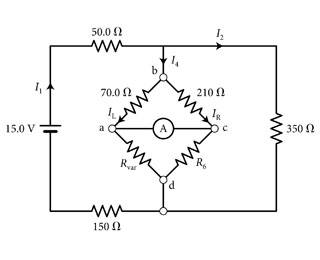# Current through left bridge of a Wheatstone Bridge

• nagyn
In summary, the ammeter in the Wheatstone bridge of Figure 1 measures zero current when the resistance Rvar of the variable resistor is set to 189 Ω.f

## Homework Statement

The ammeter in the Wheatstone bridge of (Figure 1) measures zero current when the resistance Rvar of the variable resistor is set to 189 Ω .What is the current IL through the left side of the bridge?

## Homework Equations

Junction rule:
ILeft + IRight = I4
I4 + I2 = I1

Loop rule:
ILeft(70) - IRight(210) = 0

R6 = (189)(210)/(70) = 567

## The Attempt at a Solution

Using the loop rule, ILeft = 3IRight, and using the junction rule ILeft = (3/4)I4. So I figured in order to determine ILeft I needed to find I4, and the only way to find that was to find I1 and I2.

The entire equivalent resistance of the diamond: the left and right bridges have in-series resistors, so on the left: 189+70 = 259. On the right, 210+567 = 777. The bridges themselves are in parallel, so 1/Rdiamond = (1/777) + (1/259).

Rdiamond = 194.25

Using the same approach for the rightmost branch of the entire circuit, 1/Req = (1/Rdiamond) + (1/350)

Req = 124.92

So the total resistance is Req + 50 + 150 = 324.92

I1 = V/Rtotal = 15/324.92 = 0.0462

Then this might be where I'm messing up: I tried using the loop rule again to find the ratio between I2 and I4 like I did for ILeft and IRight.

I4(194.25) - I2(350) = 0
I4 = 1.8*I2
I1 = 1.555*I4

So I4 = 0.0462/1.555 = 0.0297

And now ILeft = (3/4)I4 = (3/4)(0.0297) = 0.0223 A

This is not the correct answer. Like I said above I think I might be misunderstanding how to determine I2 and I4, but I'm not sure what the right way to approach it is. I hope my work is fairly easy to follow

Your work looks fine to me, and the answer looks good. Perhaps they're wanting it expressed as mA rather than A? Or perhaps they're quibbling about significant figures?

Your work looks fine to me, and the answer looks good. Perhaps they're wanting it expressed as mA rather than A? Or perhaps they're quibbling about significant figures?

I dropped the third sig fig and the answer was accepted. Which seems strange to me, but I guess you were right. Thank you anyways!